# Solving equations using linear interpolation

Martin McBride, 2021-04-05
Tags solving equations linear interpolation
Categories numerical methods pure mathematics

An earlier article show how to use interval bisection to solve equations of the form $f(x) = 0$.

In this article we will look at another method, linear interpolation. This involves slightly more calculations per iteration, but it usually converges more quickly so requires less iterations to obtain a result of the required accuracy.

## Example problem

We will use the same example that we used to illustrate interval bisection, solving the equation:

$$x^2 - 2 = 0$$

Again we will look for just one of the two solutions, positive $\sqrt 2$.

Linear interpolation starts in a similar way to interval bisection. We sketch a graph of the function and identify a suitable starting interval containing exactly one root: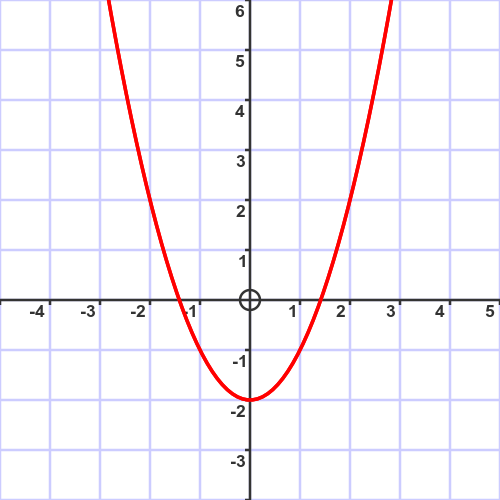As before we will start with the interval $[1, 2]$.

The process of linear interpolation proceeds as follows:

1. Start with an initial interval (1 to 2 in this case).
2. Use linear interpolation to divide the interval into new intervals.
3. Determine whether the solution is in the lower interval or the upper interval.
4. Repeat step two with the selected interval.

Steps 2 to 4 are repeated, giving a more accurate result each time, until the result is as close as you want it to be.

## A graphical explanation of linear interpolation

To gain an intuitive understanding of the process, we will go through a couple of iterations and show the results on a graph. This is for illustration only, you don't need to draw an accurate graph to use this method.

## First iteration

The first iteration starts with an interval $[1, 2]$. Here is the graph where we have zoomed in to the region of interest.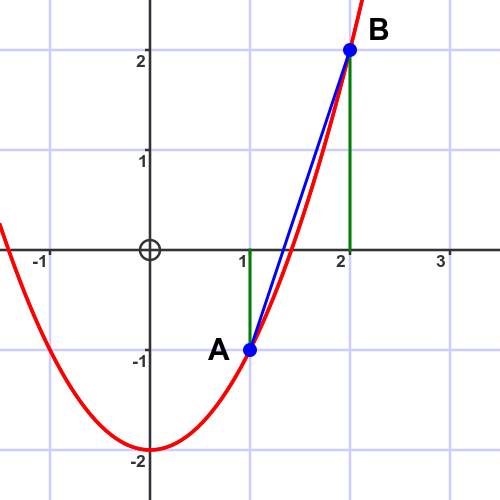Point A is the point on the curve when $x = 1$. It has coordinates (1, -1).

Point B is the point on the curve when $x = 2$. It has coordinates (2, 2).

We draw a line between points A and B (shown in blue below).

Let's say that the line crosses the x axis at $x = m$, and call the corresponding point on the curve M. This is shown here: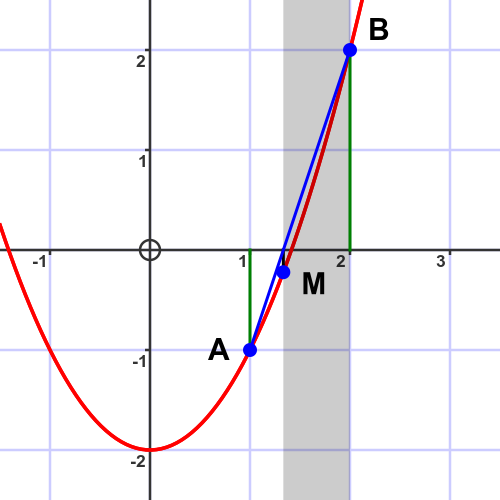In this case, M is below the x-axis and B is above the x-axis, so the solution must be between M and B.

## Second iteration

For the second iteration, we will set point A equal to the previous value of M, and repeat the same procedure. Here is the new line AB: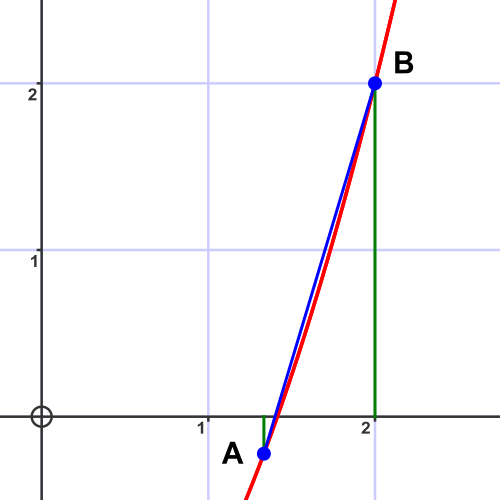And here is the new point M:This time, again, the solution is between M and B.

## Linear interpolation by calculation

An important factor that we conveniently ignored in the description above is how to calculate $m$ from A and B. Here is a diagram: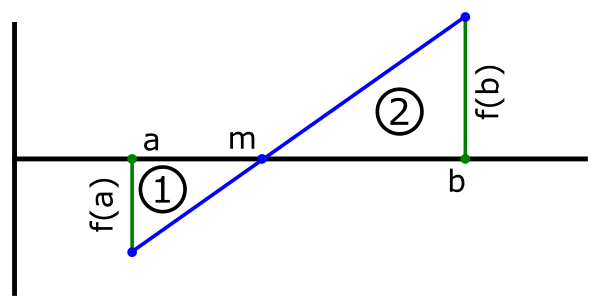Point A has an $x$ value of $a$, and similarly point B has an $x$ value of $b$.

The two triangles marked 1 and 2 are similar triangles (they are both right angled triangles, and they have the same angle at $m$). This means that their corresponding sides have equal ratios.

Triangle 1 has base $a - m$ and height $f(a)$. Triangle 2 has base $b - m$ and height $f(b)$. Since they are similar, this means that:

$$\frac {a - m}{b - m} = \frac{f(a)}{f(b)}$$

If we use the substitution:

$$r = \frac{f(a)}{f(b)}$$

We can solve for $m$:

$$m = \frac{b.r - a}{r - 1}$$

For the first iteration, we know the values of $a$ and $b$. We can calculate $f(a)$, $f(b)$, then $m$ and $f(m)$.

Here is the calculation based on $a = 1$, $b = 2$

a b f(a) f(b) m f(m)
1 2 -1 2 4/3 -2/9

Notice that $f(b)$ and $f(m)$ have opposite signs. This means that the root must be somewhere between $m$ and $b$.

For the second iteration, we therefore start with $a = 4/3$, $b = 2$ and calculate again:

a b f(a) f(b) m f(m)
4/3 2 -2/9 2 1.4 -0.04

Again, $f(b)$ and $f(m)$ have opposite signs so the root must be somewhere between $m$ and $b$.

For the third iteration, we therefore start with $a = 1.4$, $b = 2$, and so on.